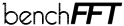Go back to the benchFFT accuracy results page.

# FFT Accuracy Benchmark Methodology

## Measurement

To compute the accuracy of an FFT, we compare the transform of uniform pseudorandom numbers in [-0.5,0.5) with the output of an arbitrary-precision arithmetic FFT (with > 40 decimal places of accuracy). This is the forward error, defined as compare(FFT(x), exactFFT(x)). We also compute the backward error, by finding the input to the arbitrary-precision FFT that gives the same output; i.e. compare(exactInverseFFT(FFT(x)), x).

To compare two arrays, we use

compare(a, b) = ||a – b||n / ||b||n
where ||x||n is the Ln norm of the vector x, defined by:
||x||n = (sum |xi|n)1/n

We compute the error for the L1, L2, and L [= max(|xi|)] norms.

For random input, the L2 forward error of a well-implemented FFT routine grows as O(√log N), where N is the size of the transform (see also our commentary). Because the Fourier transform is a unitary linear transformation, the backward L2 error is equal to the forward L2 error (at least for the complex transform). No such equality exists for the other norms. We computed the backward errors as an additional consistency check, but we do not mean to attach any particular significance to them.

On a given platform, the same random inputs are used for all transforms of the same size and type. Our benchmark program can currently measure the accuracy of 1D transforms only (both complex and real).

## Reporting

The plots on our web page contain a selection of the results and the complete, raw data are also available from our ftp site.

### Plots

In our graphs of FFT accuracy, we plot the L2 forward error for each FFT, on a log scale, as a function of size.

In order to keep the graphs readable, however, we only plot the "worst" results. That is, when a particular code has several variants (different radices, calling options, etcetera), we only plot the variant that is "on average" the least accurate (with a few exceptions, for codes of particular interest or for variants with radically different characteristics). We also only plot either the forward or the backward transform accuracy, whichever is worse "on average".

To quantify the "average" accuracy of a routine, and also to reorder the plot legend for improved readability, we define a plot rank for each FFT as follows. First, for each transform size in a plot, we compute a rank = log(error for FFT) / log(error for best FFT for that size). Then, the plot rank of a given FFT is defined as the median of its ranks for all sizes in the plot. The plot rank is only a convenient heuristic to produce useful plots, and it should not be interpreted as an absolute measure of accuracy.

### Raw data files

In the raw benchmark data output, the accuracy for all routines, for both forward and backward transforms, is collected in the file `host.accuracy` in the space-delimited format:

`name-of-code transform-type transform-size forward-L1 forward-L2 forward-L∞ backward-L1 backward-L2 backward-L∞`

where transform-type is a four-character string as described in our speed benchmark methodology page.

Go back to the benchFFT accuracy results page.